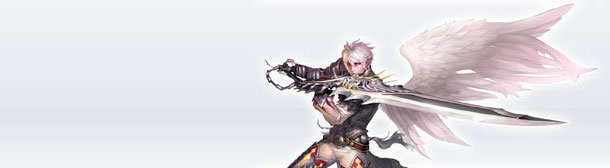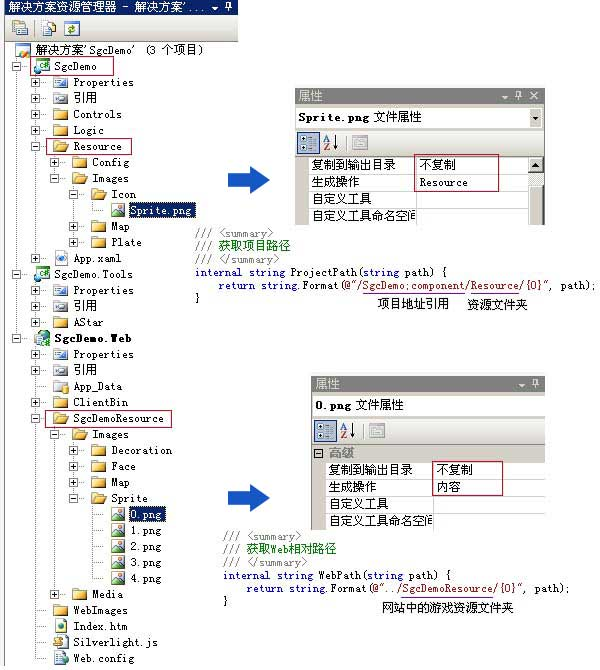/// <summary>

/// 获取项目路径

/// </summary>

internal string ProjectPath(string path) {

return string.Format(@"/SgcDemo;component/Resource/{0}", path);

}

SgcDemoResource文件夹处于SgcDemo.Web网站项目中，它主要用于存放游戏动态按需下载的资源如地图背景、背景音乐、精灵图片、魔法图片、特效装饰、头像图片等等，通过将它们的属性中的“生成操作”设置为“内容”，当Silverlight游戏制作完成后需要发布时，我们可以通过点击SgcDemo.Web项目，选择VS2008菜单中的生成->发布SgcDemo.Web即可将包含XAPSgcDemoResourceSilverlight游戏网站编译并发布到指定位置。同样的，我们可以通过如下方法获取SgcDemoResource文件夹路径：

/// <summary>

/// 获取Web相对路径

/// </summary>

internal string WebPath(string path) {

return string.Format(@"../SgcDemoResource/{0}", path);

}

/// <summary>

/// Web资源下载者

/// </summary>

/// <summary>

/// 已下载的文件路径字典

/// </summary>

static Dictionary<string, bool> files = new Dictionary<string, bool>();

/// <summary>

/// 资源下载完成时触发

/// </summary>

public event EventHandler Completed;

DispatcherTimer timer;

/// <summary>

/// 通过WebClient下载图片

/// </summary>

public void GetImage(string uri) {

//假如该路径图片还未下载过

if (!files.ContainsKey(uri)) {

WebClient webClient = new WebClient();

webClient.OpenReadCompleted += (s, e) => {

//该路径图片已下载完成

BitmapImage bitmapImage = new BitmapImage();

bitmapImage.SetSource(e.Result);

files[e.UserState.ToString()] = true;

if (Completed != null) { Completed(e.UserState, new EventArgs()); }

};

} else {

//假如该路径图片已下载完成

if (files[uri]) {

if (Completed != null) { Completed(uri, new EventArgs()); }

} else {

if (timer == null) {

//假如该路径图片正在下载(每隔1秒检测一次是否已下载完成)

timer = new DispatcherTimer() { Interval = TimeSpan.FromSeconds(1) };

timer.Tick += (s, e) => {

if (files[uri]) {

if (Completed != null) { Completed(uri, new EventArgs()); }

DispatcherTimer t = s as DispatcherTimer;

t.Stop();

t = null;

}

};

timer.Start();

}

}

}

}

}

//首先设置缩略图

map.Source = GetProjectImage(string.Format("Images/MiniMap/{0}.jpg", Code));

map.Stretch = Stretch.Fill;

//下载实际图片

map.Source = GetWebImage(string.Format("Images/Map/{0}/Background.jpg", Code));

map.Stretch = Stretch.None;

};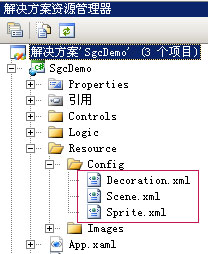<Scene Code="0">

<!--地图-->

<Map Name="荷花池" Width="1440" Height="1080" CanScroll="False" ScrollSpeed ="10" MatrixSize="32" GridSize="40" Gradient="63.4" ReferenceStyle="0" OffsetX="861" OffsetY="25" RotationX="0" CenterOfRotationX="0" RotationY="0" CenterOfRotationY="0" RotationZ="0" CenterOfRotationZ="0" Music="0" Teleport="7_6_0,7_7_0" Terrain="0_16_0,0_17_0,0_18_0,0_19_0,0_20_0,1_16_0,1_20_0,2_16_0,2_20_0,3_4_0,3_5_0,3_6_0,3_7_0,3_8_0,3_9_0,3_10_0,3_11_0,3_12_0,3_13_0,3_14_0,3_15_0,3_16_0,3_20_0,4_4_0,4_20_0,5_4_0,5_20_0,6_4_0,6_20_0,7_4_0,7_10_0,7_11_0,7_12_0,7_13_0,7_14_0,7_15_0,7_16_0,7_20_0,8_4_0,8_10_0,8_16_0,8_20_0,9_4_0,9_10_0,9_16_0,9_20_0,10_4_0,10_5_0,10_6_0,10_10_0,10_16_0,10_20_0,11_6_0,11_10_0,11_16_0,11_20_0,12_6_0,12_10_0,12_16_0,12_20_0,13_6_0,13_10_0,13_16_0,13_20_0,14_6_0,14_10_0,14_15_0,14_16_0,14_20_0,15_6_0,15_10_0,15_14_0,15_15_0,15_20_0,16_6_0,16_10_0,16_11_0,16_12_0,16_13_0,16_14_0,16_19_0,16_20_0,17_6_0,17_18_0,17_19_0,18_6_0,18_17_0,18_18_0,19_6_0,19_16_0,19_17_0,20_6_0,20_7_0,20_8_0,20_9_0,20_10_0,20_11_0,20_12_0,20_13_0,20_14_0,20_15_0,20_16_0,"/>

<!--遮挡-->

<Mask Code="0" Opacity="0.6" X="969" Y="244" Z="16"/>

<Mask Code="1" Opacity="0.6" X="926" Y="470" Z="100"/>

<Mask Code="2" Opacity="0.6" X="581" Y="556" Z="100"/>

<Mask Code="3" Opacity="0.6" X="155" Y="320" Z="100"/>

<Mask Code="4" Opacity="0.6" X="743" Y="271" Z="27"/>

<Mask Code="5" Opacity="0.6" X="505" Y="290" Z="24"/>

<!--动画部件-->

<Animations>

<Animation Code="1" Opacity="0.6" X="472" Y="0" Z="0"/>

</Animations>

<!--传送点(ID用于匹配传送二维数组,Code用于匹配Decoration.xml)-->

<Teleports>

<Teleport ID="0" Code="0" X="865" Y="285" Z="14" ToScene="1" ToX="70" ToY="68" ToDirection="1" Tip="大风山"/>

</Teleports>

<!--精灵-->

<Sprites>

<Sprite Code="2" X="4" Y="6" Direction="1"/>

<Sprite Code="1" X="10" Y="7" Direction="1"/>

<Sprite Code="2" X="1" Y="17" Direction="2"/>

<Sprite Code="3" X="2" Y="18" Direction="3"/>

<Sprite Code="4" X="11" Y="19" Direction="4"/>

<Sprite Code="3" X="14" Y="19" Direction="5"/>

<Sprite Code="2" X="16" Y="17" Direction="6"/>

<Sprite Code="0" X="19" Y="10" Direction="3"/>

</Sprites>

</Scene>

Map节点中的属性大家应该非常熟悉，是的，它们就是场景编辑器所能修改的相应参数。以制作三国策场景为例，我们首先点击“载入地图”将游戏实际地图图片导入到编辑器中，然后对照着地图分别对矩阵尺寸、倾斜度、XY偏移、地形等进行相应设置，当你感觉整个场景已能与地图背景完美吻合了，我们即可通过点击“导出场景信息”将此配置好的场景参数输出为xml文件，该文件中即包含了上述Map节点：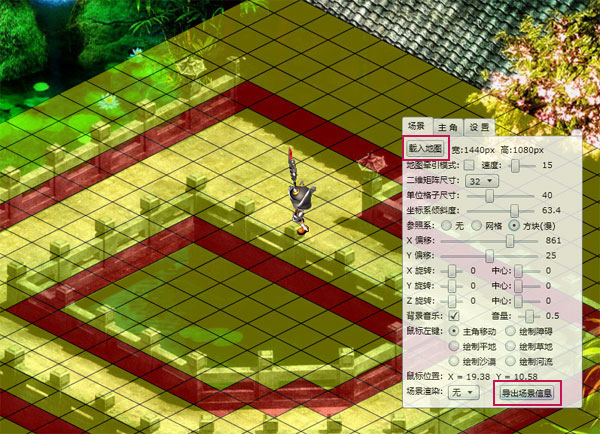enum ReferenceStyles为例，它的定义如下：

/// <summary>

/// 场景参照物样式

/// </summary>

public enum ReferenceStyles {

None = 0,

Grid = 1,

Box = 2,

}

Map节点中，我们只需将ReferenceStyle = 相应的数字(例如我要设置参照系为方块则取2)即可获得该数字对应的ReferenceStyles类型。以ReferenceStyle = 1为例，在cs中，我们只需通过ReferenceStyle = (ReferenceStyles)((int)xScene.Attribute("ReferenceStyle"));即可将该值取得并转换成代码中的枚举类型值，整个过程大致就是这样。当然，为枚举值附加数字还有更加巧妙的应用，下一节我再为大家讲解详细细节。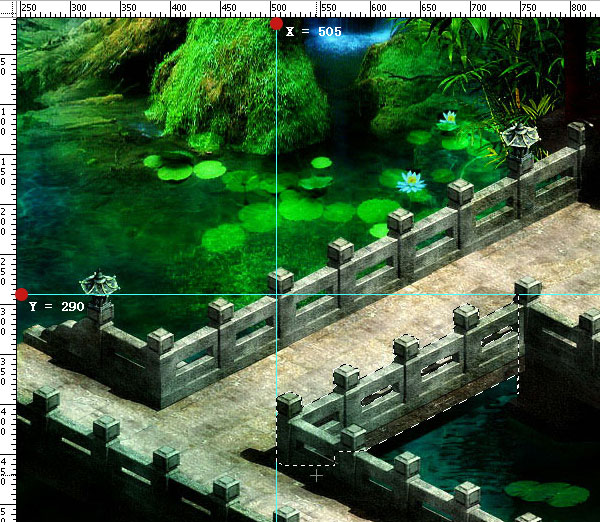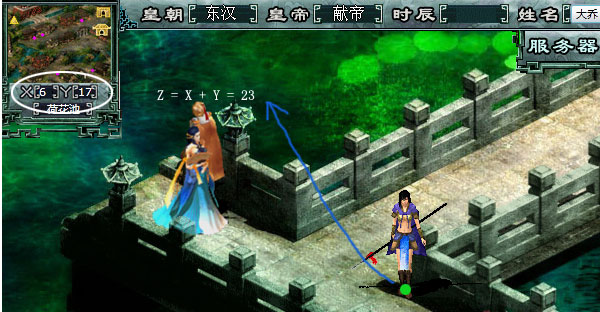Animations节点中记录的是该场景中的所有动画图，如瀑布等的位置；Teleports节点则记录的是场景中所有的传送点位置。AnimationTeleport同属于带动画的装饰物，通过它们的Code属性，类似数据库中的inner join内联到Decoration.xml数据文件，该文件中包含有该代号为Code的装饰物的详细数据，类似：

<!--装饰物-->

<Decorations>

<Decoration Code="0" Format="1" Width="960" Height="181" CenterX="60" CenterY="140" FrameNum="8" Interval="150"/>

<Decoration Code="1" Format="0" Width="1620" Height="248" CenterX="0" CenterY="0" FrameNum="9" Interval="150"/>

<Decoration Code="2" Format="0" Width="3780" Height="600" CenterX="0" CenterY="0" FrameNum="9" Interval="150"/>

</Decorations>

Sprites节点与上面的装饰物类似，包含的是场景中所有非玩家精灵所处的位置、朝向等信息，同样的通过它们的Code属性内联到Sprite.xml精灵动画图象数据文件：

<!--精灵-->

<Sprites>

<Sprite Code="0" FullName="大乔" Width="160" Height="140" Speed="280" MoveMode="1" BodyCenter="80,120" IconCenter="21,78" Frames="200, 6, 6, -1, 100, 0, 5, -1, -1, -1, -1, -1, -1, -1, -1, -1, -1, -1, -1, -1"/>

<Sprite Code="1" FullName="孙尚香" Width="72" Height="104" Speed="280" MoveMode="1" BodyCenter="33,86" IconCenter="21,78" Frames="200, 6, 6, -1, 100, 0, 5, -1, -1, -1, -1, -1, -1, -1, -1, -1, -1, -1, -1, -1"/>

<Sprite Code="2" FullName="深蓝色右手" Width="72" Height="104" Speed="280" MoveMode="1" BodyCenter="33,86" IconCenter="21,78" Frames="200, 6, 6, -1, 100, 0, 5, -1, -1, -1, -1, -1, -1, -1, -1, -1, -1, -1, -1, -1"/>

<Sprite Code="3" FullName="貂禅" Width="102" Height="136" Speed="280" MoveMode="1" BodyCenter="45,102" IconCenter="21,78" Frames="200, 6, 6, -1, 100, 0, 5, -1, -1, -1, -1, -1, -1, -1, -1, -1, -1, -1, -1, -1"/>

<Sprite Code="4" FullName="小乔" Width="128" Height="160" Speed="280" MoveMode="0" BodyCenter="61,126" IconCenter="21,78" Frames="200, 6, 6, -1, 100, 0, 5, -1, -1, -1, -1, -1, -1, -1, -1, -1, -1, -1, -1, -1"/>

</Sprites>

/// <summary>

/// 加载XML文件

/// </summary>

/// <param name="uri">XML文件地址</param>

/// <returns>XElement</returns>

}

XElement xScene = LoadXML("Scene.xml").DescendantsAndSelf("Scene").Single(X => X.Attribute("Code").Value == "0");

XElement xMap = xScene.Element("Map");

//设置相应参数

MapName = xMap.Attribute("Name").Value.ToString();

MapWidth = (double)xMap.Attribute("Width");

MapHeight = (double)xMap.Attribute("Height");

……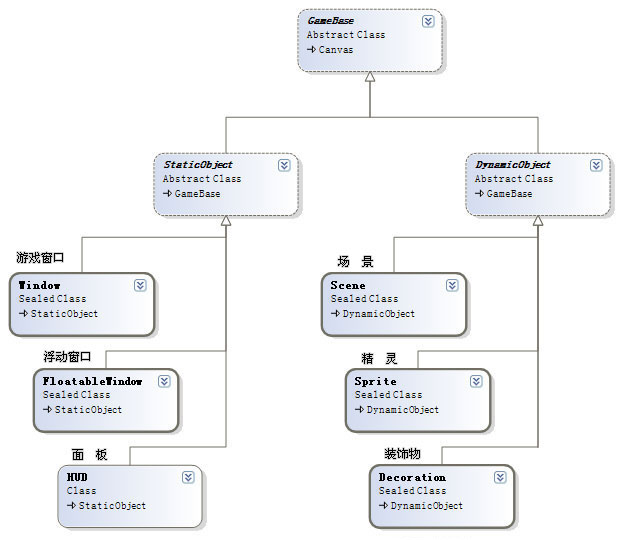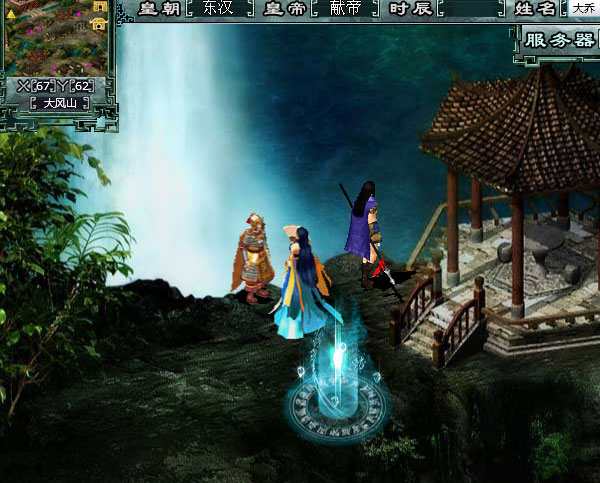posted on 2010-03-18 17:27  深蓝色右手  阅读(9151)  评论(28编辑  收藏